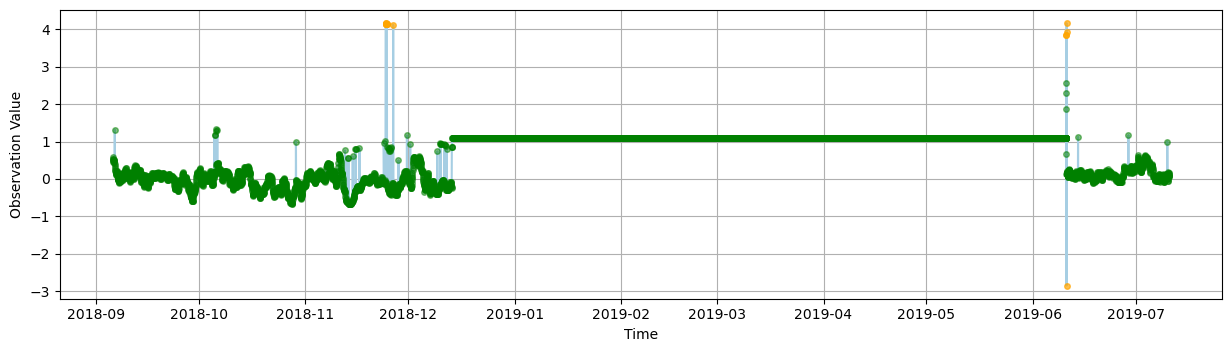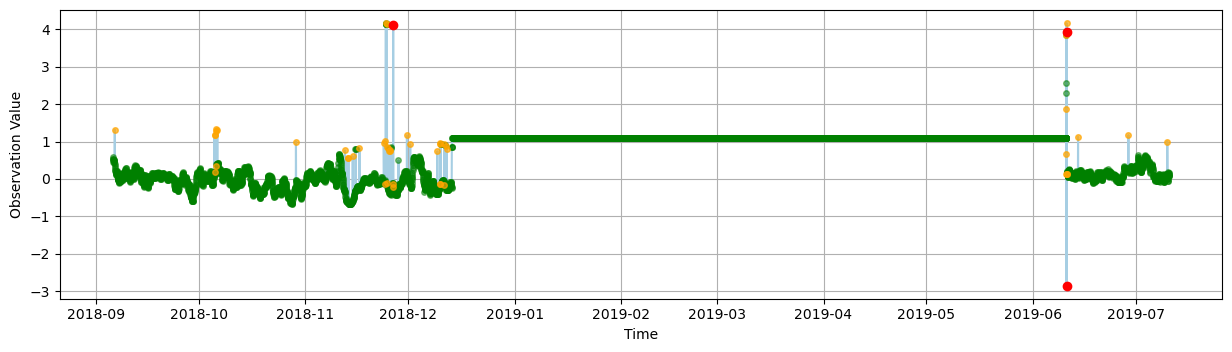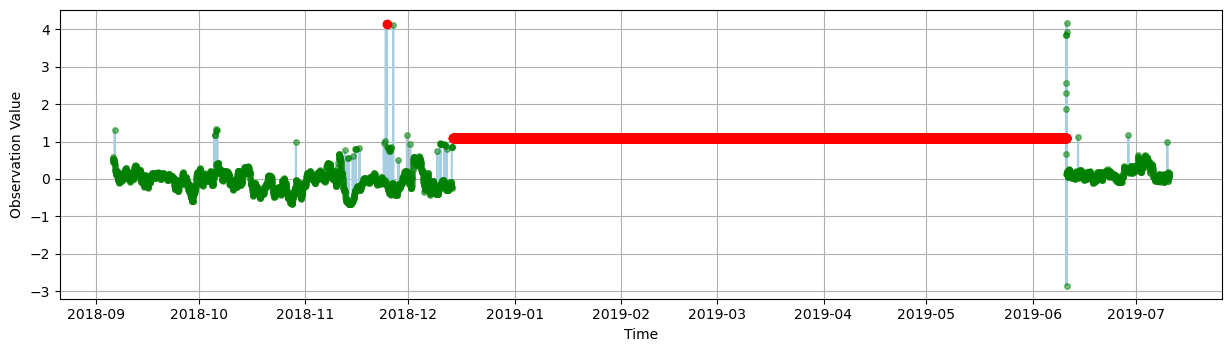# IOOS QARTOD software (ioos_qc)#

Created: 2020-02-14

Updated: 2022-05-23

This post will demonstrate how to run ioos_qc on a time-series dataset. ioos_qc implements the Quality Assurance / Quality Control of Real Time Oceanographic Data (QARTOD).

We will be using the water level data from a fixed station in Kotzebue, AK.

Below we create a simple Quality Assurance/Quality Control (QA/QC) configuration that will be used as input for ioos_qc. All the interval values are in the same units as the data.

For more information on the tests and recommended values for QA/QC check the documentation of each test and its inputs: https://ioos.github.io/ioos_qc/api/ioos_qc.html#module-ioos_qc.qartod

qc_config = {
"qartod": {
"gross_range_test": {"fail_span": [-10, 10], "suspect_span": [-2, 3]},
"flat_line_test": {
"tolerance": 0.001,
"suspect_threshold": 10800,
"fail_threshold": 21600,
},
"spike_test": {
"suspect_threshold": 0.8,
"fail_threshold": 3,
},
}
}


Now we are ready to load the data, run tests and plot results!

We will get the data from the AOOS ERDDAP server.

import cf_xarray

print(cf_xarray.__version__)
from erddapy import ERDDAP

e = ERDDAP(server="https://erddap.aoos.org/erddap/", protocol="tabledap")
e.constraints = {
"time>=": "2018-09-05T21:00:00Z",
"time<=": "2019-07-10T19:00:00Z",
}

data = e.to_xarray()

data.cf

0.8.4

Discrete Sampling Geometry:
CF Roles:   timeseries_id: ['station']

Coordinates:
CF Axes:   X: ['longitude']
Y: ['latitude']
T: ['time']
Z: n/a

CF Coordinates:   longitude: ['longitude']
latitude: ['latitude']
time: ['time']
vertical: n/a

Cell Measures:   area, volume: n/a

Standard Names:   latitude: ['latitude']
longitude: ['longitude']
time: ['time']

Bounds:   n/a

Grid Mappings:   n/a

Data Variables:
Cell Measures:   area, volume: n/a

Standard Names:   aggregate_quality_flag: ['sea_surface_height_above_sea_level_geoid_mhhw_qc_agg']
altitude: ['z']
sea_surface_height_above_sea_level: ['sea_surface_height_above_sea_level_geoid_mhhw']
sea_surface_height_above_sea_level quality_flag: ['sea_surface_height_above_sea_level_geoid_mhhw_qc_tests']

Bounds:   n/a

Grid Mappings:   n/a

from ioos_qc.config import QcConfig

qc = QcConfig(qc_config)

# The result is always a list but we only want the first, one and only in this case, variable.
variable_name = data.cf.standard_names["sea_surface_height_above_sea_level"]

qc_results = qc.run(
inp=data[variable_name],
tinp=data.cf["T"].to_numpy(),
)

qc_results

defaultdict(collections.OrderedDict,
{'qartod': OrderedDict([('gross_range_test',
array([1, 1, 1, ..., 1, 1, 1], dtype=uint8)),
('flat_line_test',
array([1, 1, 1, ..., 1, 1, 1], dtype=uint8)),
('spike_test',
array([2, 1, 1, ..., 1, 1, 2], dtype=uint8))])})


The results are returned in a dictionary format, similar to the input configuration, with a mask for each test. While the mask is a masked array it should not be applied as such. The results range from 1 to 4 meaning:

1. data passed the QA/QC

2. did not run on this data point

3. flag as suspect

4. flag as failed

Now we can write a plotting function that will read these results and flag the data.

import matplotlib.pyplot as plt
import numpy as np

def plot_results(data, variable_name, results, title, test_name):
time = data.cf["time"]
obs = data[variable_name]
qc_test = results["qartod"][test_name]

qc_pass = np.ma.masked_where(qc_test != 1, obs)
qc_suspect = np.ma.masked_where(qc_test != 3, obs)
qc_fail = np.ma.masked_where(qc_test != 4, obs)
qc_notrun = np.ma.masked_where(qc_test != 2, obs)

fig, ax = plt.subplots(figsize=(15, 3.75))
fig.set_title = f"{test_name}: {title}"

ax.set_xlabel("Time")
ax.set_ylabel("Observation Value")

kw = {"marker": "o", "linestyle": "none"}
ax.plot(time, obs, label="obs", color="#A6CEE3")
ax.plot(
time, qc_notrun, markersize=2, label="qc not run", color="gray", alpha=0.2, **kw
)
ax.plot(
time, qc_pass, markersize=4, label="qc pass", color="green", alpha=0.5, **kw
)
ax.plot(
time,
qc_suspect,
markersize=4,
label="qc suspect",
color="orange",
alpha=0.7,
**kw,
)
ax.plot(time, qc_fail, markersize=6, label="qc fail", color="red", alpha=1.0, **kw)
ax.grid(True)

title = "Water Level [MHHW] [m] : Kotzebue, AK"


The gross range test test should fail data outside the $$\\pm$$ 10 range and suspect data below -2, and greater than 3. As one can easily see all the major spikes are flagged as expected.

plot_results(
data,
variable_name,
qc_results,
title,
"gross_range_test",
)An actual spike test, based on a data increase threshold, flags similar spikes to the gross range test but also indetifies other suspect unusual increases in the series.

plot_results(
data,
variable_name,
qc_results,
title,
"spike_test",
)The flat line test identifies issues with the data where values are “stuck.”

ioos_qc succefully identified a huge portion of the data where that happens and flagged a smaller one as suspect. (Zoom in the red point to the left to see this one.)

plot_results(
data,
variable_name,
qc_results,
title,
"flat_line_test",
)This notebook was adapted from Jessica Austin and Kyle Wilcox’s original ioos_qc examples. Please see the ioos_qc documentation for more examples.